# Sequence and Series - Definition of Arithmetic Progression with example.

DEFINITION

Sequence :

A succession of terms a1, a2, a3, a4........ formed according to some rule or law.

Examples are :

1, 4, 9, 16, 25,.....

–1, 1, –1, 1,........

x, 2x, 3x, 4x,......

1!, 2!, 3!, 4!,......

A finite sequence has a finite (i.e. limited) number of terms, as in the first example above. An infinite sequence has an unlimited number of terms, i.e. there is no last term, as in the second and third.

Series

The indicated sum of the terms of a sequence. In the case of a finite sequence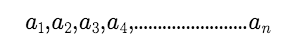the

the corresponding series isThis series has a finite or limited number of terms and is called a finite series.

2.ARITHMETIC PROGRESSION (A.P.)

A.P. is a sequence whose terms differ by a fixed number. This fixed number is called the common difference. If a is the first term & d the common difference, then A.P. can be written as

a, a + d, a + 2d, .............., a + (n – 1) d , ..........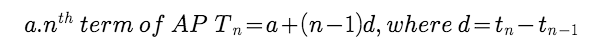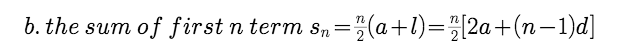where l is the last term.

Points to remember for Airthmetic Progression

1. nth term of an A.P. is of the form An + B i.e. a linear expression in 'n', in such a case the coefficient of n is the common difference of the A.P. i.e. A.

2. Sum of first 'n' terms of an A.P. is of the form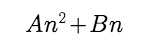i.e. a quadratic expression in 'n', in such case the common difference is twice the coefficient of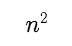. i.e. 2A

3. Also nth term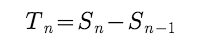Example :

If(x+1),3xand(4x+2)are first three terms of an A.P. then its 5th term is-

Solution

(x+1), 3x and (4x+2) are in AP then

3x -(x+1)=(4x+2)-3x  i.e x=3

a =4 and d = 9-4 =5

Tn = 4+ 4*5 = 24

Example :

The sum of first four terms of an A.P. is 56 and the sum of it's last four terms is 112. If its first term is 11 then find the number of terms in the A.P.

Solution :

a + a + d + a + 2d + a + 3d = 56
4a + 6d = 56
44 + 6d = 56 (as a = 11)
6d = 12 hence d = 2
Now sum of last four terms.
a + (n – 1)d + a + (n – 2)d + a + (n – 3)d + a + (n – 4)d = 112

=>  4a + (4n – 10)d = 112  44 + (4n – 10)2 = 112

=>  4n – 10 = 34

=>  n = 11

Example :

The sum of first n terms of two A.Ps. are in ratio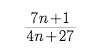. Find the ratio of their 11th terms.

Solution :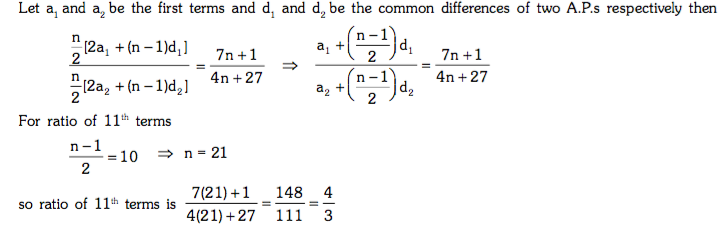Post By : Amit Rai 18 Apr, 2019 2440 views Maths